Next: Worked example 6.1: Cannon Up: Conservation of momentum Previous: Collisions in 1-dimension

## Collisions in 2-dimensions

Suppose that an object of mass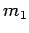, moving with initial speed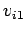, strikes a second object, of mass, which is initially at rest. Suppose, further, that the collision is not head-on, so that after the collision the first object moves off at an angle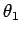to its initial direction of motion, whereas the second object moves off at an angle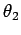to this direction. Let the final speeds of the two objects be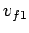and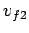, respectively. See Fig. 55.

We are again considering a system in which there is zero net external force (the forces associated with the collision are internal in nature). It follows that the total momentum of the system is a conserved quantity. However, unlike before, we must now treat the total momentum as a vector quantity, since we are no longer dealing with 1-dimensional motion. Note that if the collision takes place wholly within the-plane, as indicated in Fig. 55, then it is sufficient to equate the- and- components of the total momentum before and after the collision.

Consider the-component of the system's total momentum. Before the collision, the total-momentum is simply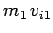, since the second object is initially stationary, and the first object is initially moving along the-axis with speed. After the collision, the-momentum of the first object is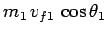: i.e.,times the-component of the first object's final velocity. Likewise, the final-momentum of the second object is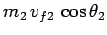. Hence, momentum conservation in the-direction yields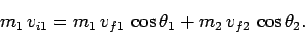(240)

Consider the-component of the system's total momentum. Before the collision, the total-momentum is zero, since there is initially no motion along the-axis. After the collision, the-momentum of the first object is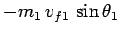: i.e.,times the-component of the first object's final velocity. Likewise, the final-momentum of the second object is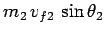. Hence, momentum conservation in the-direction yields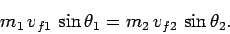(241)

For the special case of an elastic collision, we can equate the total kinetic energies of the two objects before and after the collision. Hence, we obtain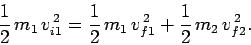(242)

Given the initial conditions (i.e.,,, and), we have a system of three equations [i.e., Eqs. (240), (241), and (242)] and four unknowns (i.e.,,,, and). Clearly, we cannot uniquely solve such a system without being given additional information: e.g., the direction of motion or speed of one of the objects after the collision.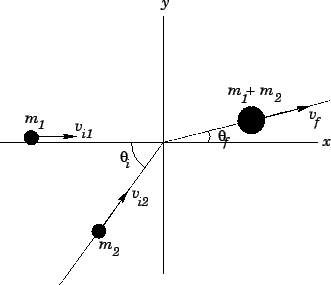Figure 56 shows a 2-dimensional totally inelastic collision. In this case, the first object, mass, initially moves along the-axis with speed. On the other hand, the second object, mass, initially moves at an angle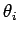to the-axis with speed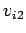. After the collision, the two objects stick together and move off at an angle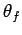to the-axis with speed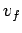. Momentum conservation along the-axis yields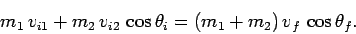(243)

Likewise, momentum conservation along the-axis gives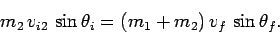(244)

Given the initial conditions (i.e.,,,,, and), we have a system of two equations [i.e., Eqs. (243) and (244)] and two unknowns (i.e.,and). Clearly, we should be able to find a unique solution for such a system.Next: Worked example 6.1: Cannon Up: Conservation of momentum Previous: Collisions in 1-dimension
Richard Fitzpatrick 2006-02-02